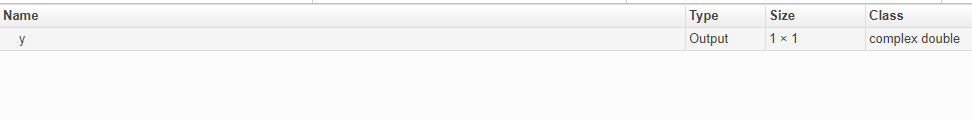## 复数数据的代码生成

### 定义复变量时的限制

```x = 5 + 6i; % x is a complex number by assignment. y = complex(5,6); % y is the complex number 5 + 6i. ```

```function x = test1( ) x = zeros(3,3); % x is real for k = 1:numel(x) x(k) = 3 + 4i; end end```

```function x = test1( ) x = zeros(3,3)+ 0i; %x is complex for k = 1:numel(x) x(k) = 3 + 4i; end end```

### 具有零值虚部的复数数据的代码生成

• 在某些情况下，按绝对值对复数数据排序的函数所生成的结果可能与 MATLAB® 结果不同。请参阅按绝对值对复数值排序的函数

• 对于要求按绝对值对复数输入排序的函数，具有零值虚部的复数输入必须按绝对值排序。这些函数包括 `ismember``union``intersect``setdiff``setxor`

#### 生成的复数参数 MEX 函数的输入和输出

• 假设您生成使用复数输入的 MEX 函数。如果使用实数输入调用该 MEX 函数，MEX 函数会将实数输入转换为具有零值虚部的复数值。

• 如果 MEX 函数返回虚部均为零值的复数值，则 MEX 函数将这些值以实数值返回到 MATLAB 工作区。以如下函数为例：

```function y = foo() y = 1 + 0i; % y is complex with imaginary part equal to zero end ```

如果您为 `foo` 生成一个 MEX 函数并查看代码生成报告，会看到 `y` 是复数。

`codegen foo -report`如果运行该 MEX 函数，可以看到在 MATLAB 工作区中，`foo_mex` 的结果是实数值 `1`

`z = foo_mex `
```ans = 1```

### 含复数操作数的表达式的结果

```z = x + y; ```

• 当复数结果的虚部为零时，MEX 函数将结果以实数值返回到 MATLAB 工作区。请参阅生成的复数参数 MEX 函数的输入和输出

• 当参数的虚部为零时，外部函数的复数参数是实数。

```function y = foo() coder.extrinsic('sqrt') x = 1 + 0i; % x is complex y = sqrt(x); % x is real, y is real end ```
• 使用复数参数但产生实数结果的函数会返回实数值。

```y = real(x); % y is the real part of the complex number x. y = imag(x); % y is the real-valued imaginary part of x. y = isreal(x); % y is false (0) for a complex number x. ```
• 接受实数参数但结果为复数的函数会返回复数值。

```z = complex(x,y); % z is a complex number for a real x and y. ```

### 具有非有限值的复数乘法的结果

• 复数值乘以复数值 (a + bi) (c + di) 定义为 (ac - bd) + (ad + bc)i。即使实部或虚部为零，也会执行完整的计算。

• 实数值乘以复数值 c(a + bi) 定义为 ca + cbi